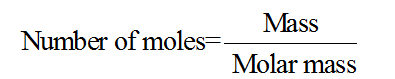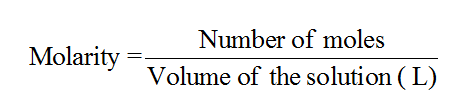# A solution contains 4.25g of ammonia per 250 mL of solution.Electrical conductivity measurements at 25 C show that 42 %of theammonia has reacted with water. Write the equation for this reaction andcalculate the pH of the solution.

Question
1 views

A solution contains 4.25g of ammonia per 250 mL of solution.
Electrical conductivity measurements at 25 C show that 42 %of the
ammonia has reacted with water. Write the equation for this reaction and
calculate the pH of the solution.

check_circle

Step 1

The number of moles for a given compound or a molecule refers to the ratio of its mass to the molecular mass. The mathematical relationship is as follows:Step 2

The molarity of the given chemical solution is related to the number of moles of the solute and the volume of the solution (in liters) as follows:Step 3

The pH of a given solution can be termed as a measure of its basicity or acidity. It is a measure of the concentration of the hydrogen or the hydronium ions which are present in the given solution. More the pH of a given solution, more will be its basicity, and lesser the pH of a given solution, more will be its acidity.

&nbs...

### Want to see the full answer?

See Solution

#### Want to see this answer and more?

Solutions are written by subject experts who are available 24/7. Questions are typically answered within 1 hour.*

See Solution
*Response times may vary by subject and question.
Tagged in